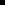#### Timestamp Calculation

The definition of the COR_STAMP structure is::

typedef struct cor_time_stamp {

COR_I4   yyyymmdd;

COR_I4   hhmmsstt;

} COR_STAMP;

where COR_I4 is a four-byte integer.

For example, for the date March 4, 1995 and time 05:06:07.08, the COR_STAMP structure looks like this:

19950304

05060708

To save the date or time timestamp in Registers, you will need to do the following:Divide the 8-byte date or time by 65536 and put the quotient in the high order timestamp register.Multiply the quotient by 65536, subtract the result from the original number and put the result in the low order timestamp register.

For example, to place the timestamp of March 4, 1995 05:06:07.08 in Register memory starting at %R518:

%R519 = 19950304 / 65536 = 304

%R518 = 19950304 - (304*65536) = 19950304 - 19922944 = 27360

%R521 = 05060708 / 65536 = 77

%R520 = 05060708 - (77*65536) = 05060708 - 05046272 = 14436

 Unsolicited compound messages with timestamps.